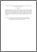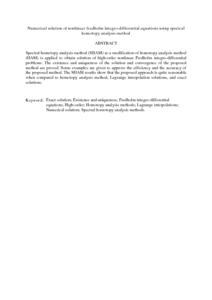# Numerical solution of nonlinear fredholm integro-differential equations using spectral homotopy analysis method

## Citation

Atabakan, Z. Pashazadeh and Nasab, A. Kazemi and Kilicman, Adem and Eshkuvatov, Zainidin K. (2013) Numerical solution of nonlinear fredholm integro-differential equations using spectral homotopy analysis method. Mathematical Problems in Engineering, 2013. art. no. 674364. pp. 1-9. ISSN 1024-123X

## Abstract

Spectral homotopy analysis method (SHAM) as a modification of homotopy analysis method (HAM) is applied to obtain solution of high-order nonlinear Fredholm integro-differential problems. The existence and uniqueness of the solution and convergence of the proposed method are proved. Some examples are given to approve the efficiency and the accuracy of the proposed method. The SHAM results show that the proposed approach is quite reasonable when compared to homotopy analysis method, Lagrange interpolation solutions, and exact solutions.Preview
PDF (Abstract)
Numerical solution of nonlinear fredholm integro.pdfView Item